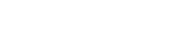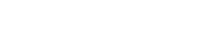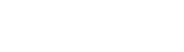# Differential Pressure Flow Meters

Differential Pressure Flow Meter is also called DP flow meter. Differential pressure flow meters use the principle of differential pressure to measure the flow of liquid, gas and steam.

The differential pressure flow meter is measured by the working principle that there is a certain relationship between the pressure difference and the flow rate when the medium fluid flows through the throttling device. Differential pressure (DP) flow meters are the most widely used form of flow measurement products today. Including: integrated orifice flowmeter, average velocity tube flowmeter, venturi tube, wedge flowmeter, underwater flowmeter, orifice plate, orifice carrier assembly, nozzle flowmeter.

Sino-Inst offers a variety of  DP flow meters for flow measurement. If you have any questions, please contact our sales engineers.

## Differential Pressure Flow Meters Applications

Extended Reading: Differential Pressure (DP) Flow Meters Technology

1. Wide range of applications. There is no other type of flowmeter comparable to a differential pressure flowmeter.
2. The orifice plate is the most widely used differential pressure flow meter. It has a firm structure, stable and reliable performance and long service life.
3. The detection parts, differential pressure transmitter, and flow totalizer can be produced by different manufacturers. It is convenient for scale economic production

1. The measurement accuracy of differential pressure flow meters is generally low.
2. The range is narrow, generally only 3:1-4:1
3. Different pressure flow meters require high on-site installation conditions.
4. The pressure loss of orifice plate and nozzle is large.

## Differential pressure flow meter working principle

When the fluid flows through the throttle, the fluid will be accelerated due to the fluid being compressed.

As the velocity of the fluid increases, the kinetic energy of the fluid will increase accordingly.

According to the law of conservation of energy, we know that the static pressure of the fluid being accelerated will reduce the corresponding value.

As the picture shows:

The cross-sectional area of the pipe at cross-section 1 is A1. The average flow velocity of the fluid when flowing through the cross section A1 is V1. Its density is ρ1.

When the fluid flows through cross section 2. The average flow rate of the fluid becomes V2. The density is ρ2. The cross-sectional area is A2.

According to the principle of fluid flow continuity, there is the following relationship: V1×A1×ρ1=V2×A2×ρ2.

If the fluid is a liquid, the density of the fluid before and after compression is generally considered constant. That is, ρ1=ρ2=ρ.

Therefore, the volumetric flow rate of the liquid: qv=V1×A1=V2×A2 ——–Formula①

According to the Bernoulli equation (i.e. the law of conservation of energy), Z1=Z2 on a horizontal pipe, then the following relationship is given:—————Formula②

Applying the Bernoulli equation and the principle of flow continuity, there are the following relations on the two cross sections:——————Formula ③

From formula ①, we get: V1=A2/A1×V2; put formula ① and formula ② into formula ③ to get:———————Formula④

In the above formula: A1=(πD2)/4, A2=(πd2)/4, according to the definition of the diameter ratio according to the diameter ratio β: β=d/D. From formula ①, we can get: V2=qv/A2

Therefore: The theoretical flow formula can be derived from this:————————–Formula ⑤

Also defined by the outflow coefficient C is: C=actual flow/theoretical flow,
Finally, the calculation formula of differential pressure flowmeter can be obtained:————————-Formula ⑥;

The expansibility coefficient of ε measured medium in formula ⑥, generally speaking ε=1 for liquid.

It can compress fluid ε<1 for gas, steam, etc.
qv is the volume flow of the fluid, unit: m3/s).
ρ1 is the density of the fluid upstream of the throttle (front) under working conditions, in kg/m3.
d is the equivalent opening diameter of the throttle under working conditions, unit: m.
△P is differential pressure, △P=P1-P2, unit: Pa.

## Differential pressure flowmeter selection

The selection of differential pressure flowmeter should consider the following aspects:

1.Accuracy, repeatability, linearity, flow range and range

The standard parts of the differential pressure flowmeter have a strict range of use. These parameters include pipe diameter ratio, Reynolds coefficient, pipe wall thickness and other parameters.

Therefore, these series of parameters should be selected reasonably in actual selection and application. In addition, the accuracy of the differential pressure flowmeter depends to a large extent on the conditions of use on site, and generally depends on the conditions of the fluid.

2.Fluid characteristics

The characteristics of the fluid refer to the fluid’s medium conditions such as density, dynamic viscosity, pressure, temperature, corrosivity, abrasion, fouling, and dirt.
Therefore, the selection is a differential pressure flowmeter that should be reasonably selected according to the physical characteristics of the fluid medium on site. If you do not understand the physical characteristics of the fluid on site, you should actively use the instrument to measure these physical parameters.

3.Economic factors

Should consider the purchase cost of differential pressure flowmeter. Installation costs and after installation. Later maintenance costs. Calibration fee. The later operating cost of the larger diameter differential pressure flowmeter is a relatively large expense. Therefore, the proper caliber differential pressure flowmeter should be selected when selecting the model.

4.Pressure loss

Large pressure loss is one of the main disadvantages of differential pressure flow meters. Orifice plates and nozzles in various throttling devices are throttling parts with large pressure loss. At the same flow rate and β value, the pressure loss of the nozzle is only 30%-50% of the pressure loss of the orifice, which means that the nozzle has a lower pressure loss.

Various flow tubes (Venturi tube, Dole tube, Rollos tube, general Venturi tube, etc.) are throttling devices with low pressure loss. Their pressure loss is only 20% of the orifice plate, even as low as 5%-10%. The development and application of these throttling devices is a direction for future efforts.

Differential pressure flow meters are one of the most widely used types of flow meters. Sino-Inst analyzed the working principle and selection of differential pressure flow meters. It provides a certain theoretical reference basis for the selection and use of differential pressure flow meters in the future.

You may like: